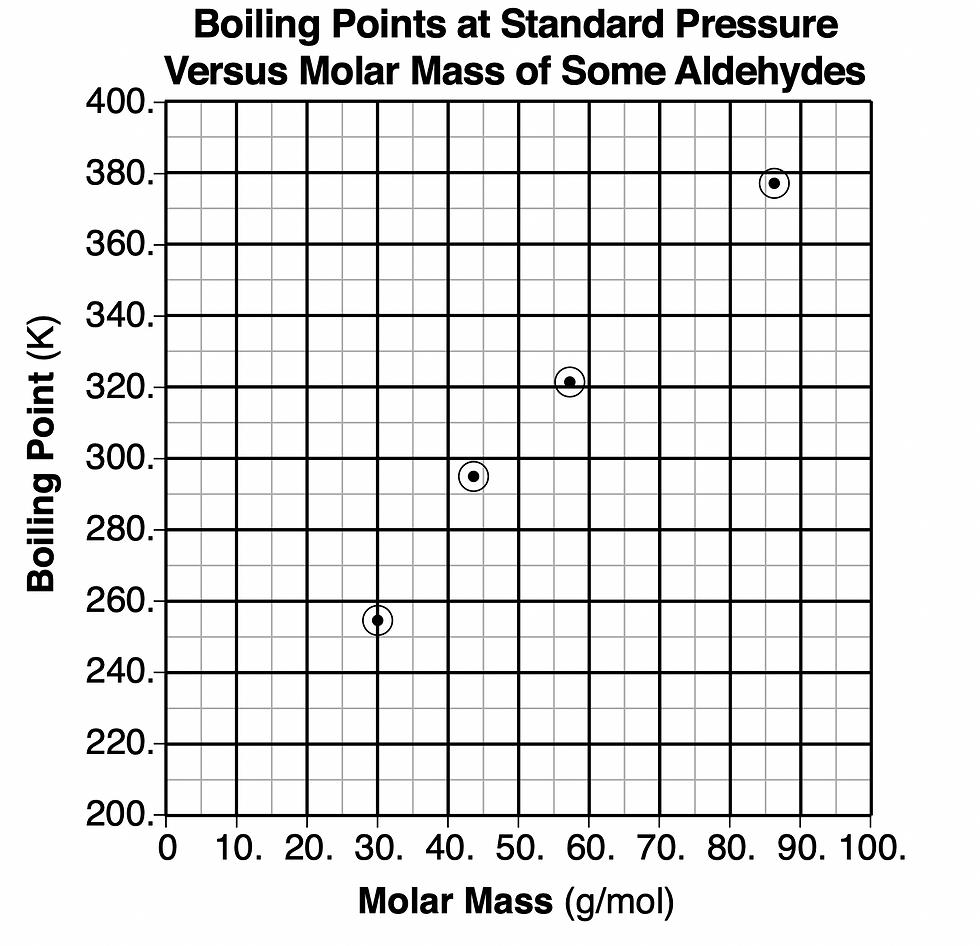top of page
Search

# The table and graph below show information about five aldehydes.

Regents Chemistry Exam August 2022

The table and graph below show information about five aldehydes.58. Based on the graph, determine the boiling point of butanal at standard pressure. 

Butanal has a molar mass of 72.1 according to the first chart. We need to trace the molar mass in the second graph on the x axis and go up on the graph. We can connect the dots to see the line for all the boiling points and see where the boiling point for 72.1 molar mass would fall.

Answer: Somewhere between 340 and 360 K

59. Determine the mass of 3.00 moles of propanal using the molar mass given in the table. 

The molar mass of propanal is 58.1 g/mol according to the first chart. We need to convert moles to mass.

We can use Table T to find the formula for the conversion which is

number of moles = given mass/ gram formula mass.

Now we can plug in: 3.00 moles = mass/ 58.1 g/mol